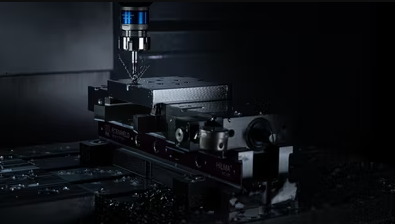## Insurance Analysis

A machine shop recently bought a new CNC mill, and is performing a risk and cost analysis to determine if insurance is necessary. The shop paid $100,000 for the tool. The annual cost for the insurance premium is$2,000, with a $1,000 deductible. After some initial analysis, the risk options to purchase or decline insurance are as follows: • 88% chance of no accident • 11% chance of a small$800 accident
• 1% chance of a total loss for the CNC mill
Should the machine shop purchase insurance? Based on that decision, what is the max projected cost savings potential?Hint
In a benefit-cost analysis, the benefits $$B$$ of a project should exceed the estimated costs $$C$$ .
$$B-C\geq 0\; or \; \frac{B}{C}\geq 1$$$Hint 2 Expected Value: $$EV=\sum (cost*probability)$$$
In a benefit-cost analysis, the benefits $$B$$ of a project should exceed the estimated costs $$C$$ .
$$B-C\geq 0\; or \; \frac{B}{C}\geq 1$$$Expected Value is: $$EV=\sum (cost*probability)$$$
Let’s first determine the projected total cost with insurance, then compare it to the projected total cost without insurance.
$$EV_{insurance}=(Cost_{1}Prob_{1})+(Cost_{2}Prob_{2})+(Cost_{3}Prob_{3})$$$$$=(\0\times 0.88)+(\800\times 0.11)+(\1,000\times 0.01)$$$
$$=\0+\88+\10=\98$$$Total annual cost with insurance premium:$2,000 + $98 =$2,098

$$EV_{no\:insurance}=(Cost_{1}Prob_{1})+(Cost_{2}Prob_{2})+(Cost_{3}Prob_{3})$$$$$=(\0\times 0.88)+(\800\times 0.11)+(\100,000\times 0.01)$$$
$$=\0+\88+\1,000=\1,088$$$Total annual cost out of pocket:$1,088

Comparing the Expected Value with insurance to no insurance:
$2,098 -$1,088 = $1,010 savings by going with no insurance.$1,010 savings by going with no insurance.